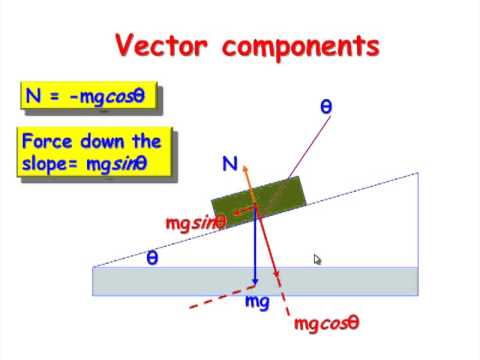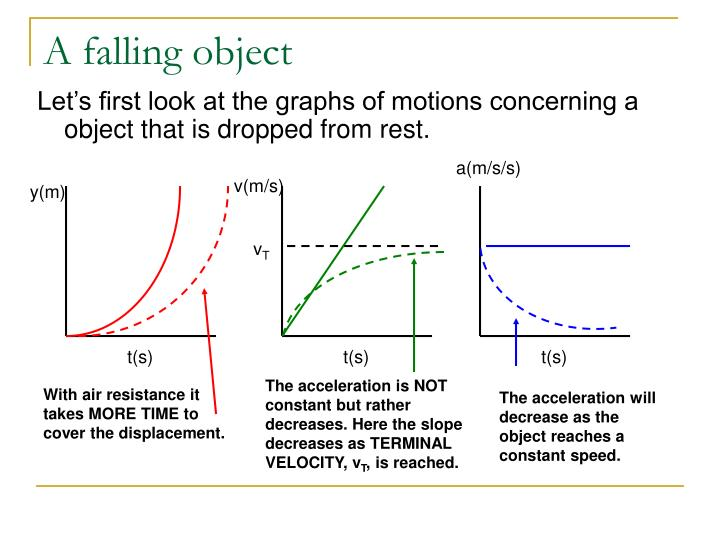# PHY C4: Weight & Falling Bodies

Here’s what you need to know:

• Weight
• Air Resistance
• Upthrust
• Drag Force

Let’s dive in!

What is Weight?
The force acting on a mass by the attraction of a gravitational field.

Since weight is a force, the equation F = ma applies.
In this case, the F is W (weight), & the ‘a’ is a constant depending on the strength of a gravitational field.
On earth, the constant is g = 9.81 ms-2.

Thus,
W = mg
In units, Newtons (N)

How does weight affect motion?
Any object with mass has weight.
An object at rest on a surface also has weight (as long as it’s in a gravitational field, e.g. around the Earth).
Thus, since the object is at rest, there MUST be another force acting in the opposite direction of the weight.
This force is called the NORMAL REACTION FORCE (R) or normal CONTACT force.
It is the force exerted by a surface onto an object resting upon it.

R acts PERPENDICULARLY (hence ‘normal’) to the plane of contact.
If the plane is parallel to level ground, the R is equal to the weight of the object:If the object is on an incline, R is equal to the COMPONENT of the weight perpendicular to the inclined plane:In Free Fall (where air resistance is negligible), this weight is the only force acting on the object.
For an object falling through air, weight is one force that acts downwards, against the forces of air resistance.

What is Air Resistance?
Resistance to motion provided by air molecules, against the direction of motion of a body.
The NET force of air resistance stems from 2 main forces:
Upthrust & Drag Force.

What is Upthrust?
We’ll learn the more general definition of upthrust in the next chapter.

In terms of an object falling through air,
Upthrust is the weight of air displaced by the object.
Just like how objects floating in water experience upthrust (buoyant force),
objects in air do too.

This is usually not very visible, since most objects have a much higher density than air,
but you can see the clear effects in balloons for example.

What is Drag Force?
Opposing force due to air molecules flowing over the body.
When air molecules collide with the moving object, they exert forces (due to their change in momentum).
The higher the velocity of these collisions, the higher the momentum of the air particles.
Thus, drag force increases as velocity of the object increases*.
At a certain velocity, the drag force will become equal to the weight of the object.
Thus, the object reaches a CONSTANT TERMINAL VELOCITY.*Interestingly, it is proportional to the square of the velocity of the object.

How does Air Resistance affect a free-falling object?

 Acceleration Initially, acceleration is g (9.81) It then decreases with time Acceleration will eventually reach 0. Velocity Initially, velocity increases (at a DECREASING rate) When a = 0, velocity is constant. This velocity is known as TERMINAL VELOCITY Displacement Initially, displacement increases (at an INCREASING rate) When v = constant, displacement increases at a constant rate## 2 thoughts on “PHY C4: Weight & Falling Bodies”

1. Pingback: PHY C4: Dynamics – ProDuckThieves

2. Pingback: PHY C4: Momentum – ProDuckThieves